Win up to 100% scholarship on Aakash BYJU'S JEE/NEET courses with ABNAT Win up to 100% scholarship on Aakash BYJU'S JEE/NEET courses with ABNAT

# Dynamics of Rigid Bodies formulae for NEET

The rigid body is an idealization of a solid body where the deformations occurring on the body are neglected. In other words, the distance between any two given points of a rigid body remains a constant regardless of the external force acting upon it. The two types of motion a rigid body can undergo are;

• Translational Motion
• Rotational Motion

### Recommended Video:

Rigid Body Dynamics Class 11 Physics One Shot – Important Questions & Concepts | NEET 2022 Physics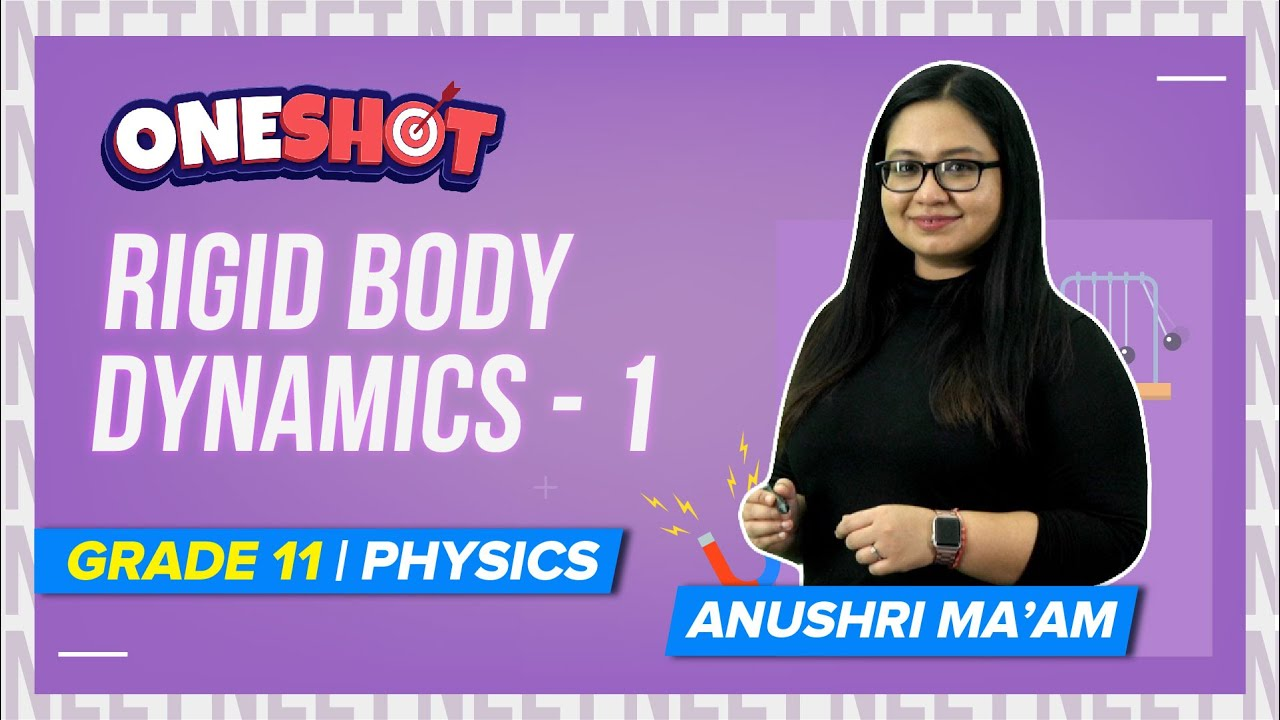### Moment of Inertia

Moment of Inertia is defined as the capacity of the system to oppose the change produced in the rotational motion of the body.

For a single particle moment of inertia I=mr2

Here m is the mass of the particle and r is the perpendicular distance from the axis about which moment of inertia is to be calculated.

For many particles, I = miri2

### Moment of Inertia of different objects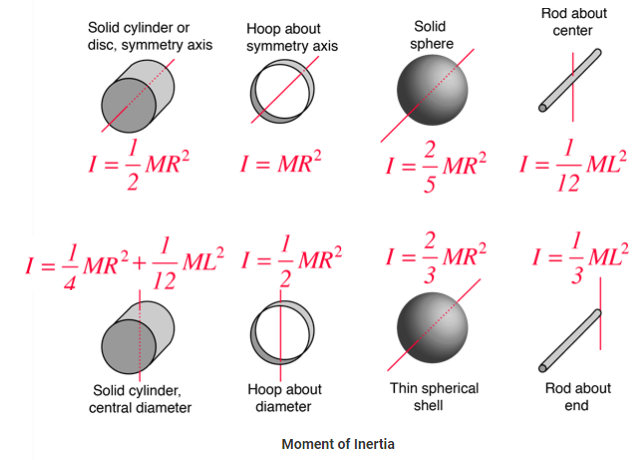K = √I/M

Here

M is the mass of the rotating object

I is the moment of inertia

### Relation Between Torque and Moment of Inertia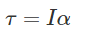τ is the torque (twisting effect of force)

I is the moment of inertia

α is the angular acceleration ( the rate of change of angular velocity)

##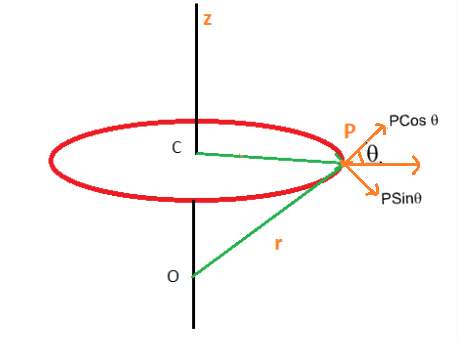Point object:  For an object accelerating around a fixed point. For example, Earth revolving around the sun. Here the angular momentum is given by:

$$\begin{array}{l}\vec{L} = r \times \vec{p}\end{array}$$

L is the angular velocity

p is the linear momentum

Extended object: The object, which is rotating about a fixed point. For example, Earth rotating about its axis. Here the angular momentum is given by

$$\begin{array}{l}\vec{L} = r \times \vec{\omega }\end{array}$$

ω is the angular velocity

L = angular momentum of the object

I = Moment of inertia

### Relation between angular momentum and Torque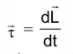Torque is the change in angular momentum

Check more:

### Recommended Video:

Friction Class 11 Physics | NEET 2022 Exam